# KSEEB SSLC Class 10 Maths Solutions Chapter 11 Introduction to Trigonometry Ex 11.3

In this chapter, we provide KSEEB SSLC Class 10 Maths Solutions Solutions Chapter 11 Introduction to Trigonometry Ex 11.3 for English medium students, Which will very helpful for every student in their exams. Students can download the latest KSEEB SSLC Class 10 Maths Solutions Solutions Chapter 11 Introduction to Trigonometry Ex 11.3 pdf, free KSEEB SSLC Class 10 Maths Solutions Solutions Chapter 11 Introduction to Trigonometry Ex 11.3 pdf download. Now you will get step by step solution to each question.

### Karnataka State Syllabus Class 10 Maths SolutionsChapter 11 Introduction to Trigonometry Ex 11.3

Question 1.
Evaluate :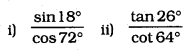iii) cos 48° – sin 42°
iv) cosec 31° – sec 59°
Solution: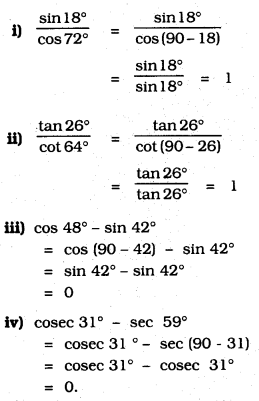Question 2.
Show that
i) tan 48° tan 23° tan 42° tan 67° = 1
ii) cos 38° cos 52° – sin 38° sin 52° = 0.
Solution:
i) tan 48° tan 23° tan 42° tan 67° = 1
LHS = tan 48° tan 23° tan 42° tan 67°
= tan 48° × tan 23° × tan (90 – 48).tan (90 – 23)
= tan 48° × tan 23° × cot 48° × cot 23°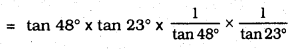= 1
∴ LHS = RHS

ii) cos 38° cos 52° – sin 38° sin 52° = 0
cos 38°. cos 52 – sin 38° sin 52
= cos 38° . cos52 – sin(90 – 52°) sin (90 – 38°)
= cos38° . cos52 – cos52 cos32°
= 0

Question 3.
If tan 2A = cot (A – 18°), where 2A is an acute angle, find the value of A.
Solution:
Since, tan 1A = cot (A – 18°)
Also, tan (2A) = cot (90° – 2A) [∵ tan θ = cot (90° – θ)]
∴ A – 18° = 90° – 2A
⇒ A + 2A = 90° + 18°
⇒ 3A = 108° ⇒ A = 108∘3 = 36°

Question 4.
If tan A = cot B, prove that A + B = 90°.
Solution:
tan A = cot B
tan A = tan (90 – B)
A = 90 – B
∴ A + B = 90°.

Question 5.
If sec 4A = cosec (A – 20°), where 4A is an acute angle, find the value of A.
Solution:
sec 4A = cosec (A – 20°)
Also, sec 4A = cosec (90° – 4A) [ ∵ cosec (90° – θ) = sec θ]
∴ A – 20° = 90° – 4A
⇒ A + 4A = 90° + 20°
⇒ 5A = 110° ⇒ A = 110∘5 = 22°

Question 6.
If A, B, and C are interior angles of a triangle ABC, then show that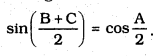Solution:
A + B + C = 180°
B + C = 180 – A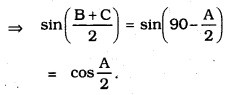Question 7.
Express sin 67° + cos 75° in terms of trigonometric ratios of angles between 0° and 45°.
Solution:
Since, sin 67° = sin (90° – 23°) = cos 23° [ ∵ sin (90° – θ ) = cos θ]
Also, cos 75° = cos (90° – 15°) = sin 15° [∵ cos (90° – θ) = sin θ]
∴ sin 67° + cos 75° = cos 23° + sin 15°

All Chapter KSEEB Solutions For Class 10 Maths

—————————————————————————–

All Subject KSEEB Solutions For Class 10

*************************************************

I think you got complete solutions for this chapter. If You have any queries regarding this chapter, please comment on the below section our subject teacher will answer you. We tried our best to give complete solutions so you got good marks in your exam.

If these solutions have helped you, you can also share kseebsolutionsfor.com to your friends.

Best of Luck!!